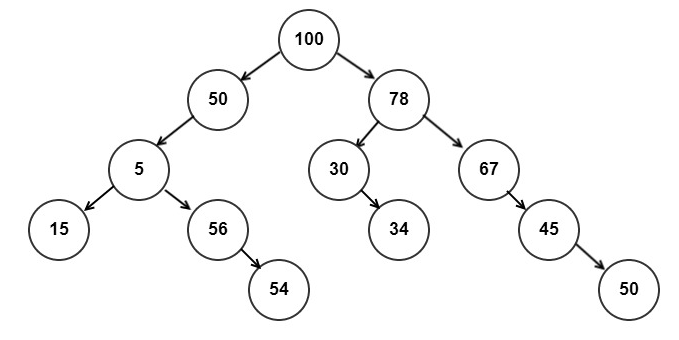Saturday, October 19, 2013

LCA for a binary tree

Given a binary tree(not a binary search tree) find the LCA of the 2 given nodes.

The lowest common ancestor(LCA) for the nodes u and v is defined as the lowest node w in tree  that has both u and v as the descendants (where a node can be a descendant of itself).

Approach:

Lets consider the below treeLCA of node 30, 5 is 100
LCA of node 15, 50 is 50
and so on

Its easy to visualize that the bottom up approach is the way to go.
Populate from the bottom to up the number of nodes matched with the target nodes and the first level to find both the nodes is the required LCA.

The short code snippet which finds the LCA of the two nodes is given below

```int lca(struct tree * root, struct tree * p, struct tree * q, struct tree  *& res) {
if(root == NULL || res != NULL)
return 0;
int found = 0;
if(root == p || root == q)
found = 1;
found += lca(root->left, p, q, res);
found += lca(root->right, p, q, res);
if(res == NULL && found == 2)
res = root;
return found;
}```